# Using 2D Contour Plots within {ggplot2} to Visualize Relationships between Three Variables

Guest post by John Bellettiere, Vincent Berardi, Santiago Estrada

The Goal

To visually explore relations between two related variables and an outcome using contour plots. We use the contour function in Base R to produce contour plots that are well-suited for initial investigations into three dimensional data. We then develop visualizations using ggplot2 to gain more control over the graphical output. We also describe several data transformations needed to accomplish this visual exploration.

The Dataset

The mtcars dataset provided with Base R contains results from Motor Trend road tests of 32 cars that took place between 1973 and 1974. We focus on the following three variables: wt (weight, 1000lbs), hp (gross horsepower), qsec (time required to travel a quarter mile). qsec is a measure of acceleration with shorter times representing faster acceleration. It is reasonable to believe that weight and horsepower are jointly related to acceleration, possibly in a nonlinear fashion.

##                    mpg cyl disp  hp drat    wt  qsec vs am gear carb
## Mazda RX4         21.0   6  160 110 3.90 2.620 16.46  0  1    4    4
## Mazda RX4 Wag     21.0   6  160 110 3.90 2.875 17.02  0  1    4    4
## Datsun 710        22.8   4  108  93 3.85 2.320 18.61  1  1    4    1
## Hornet 4 Drive    21.4   6  258 110 3.08 3.215 19.44  1  0    3    1
## Hornet Sportabout 18.7   8  360 175 3.15 3.440 17.02  0  0    3    2
## Valiant           18.1   6  225 105 2.76 3.460 20.22  1  0    3    1

Preliminary Visualizations

To start, we look at a simple scatter plot of weight by horsepower, with each data point colored according to quartiles of acceleration. We first create a new variable to represent quartiles of acceleration using the cut and quantile functions.

mtcars\$quart <- cut(mtcars\$qsec, quantile(mtcars\$qsec))

From here, we use ggplot to visualize the data. We selected colors that were sequential and color blind friendly using ColorBrewer and manually added them to the scale_colour_manual() argument within the ggplot() call below. Labels were also manually added to improve interpretation.

ggplot(mtcars, aes(x = wt, y = hp, color = factor(quart))) +
geom_point(shape = 16, size = 5) +
theme(legend.position = c(0.80, 0.85),
legend.background = element_rect(colour = “black”),
panel.background = element_rect(fill = “black”)) +
labs(x = “Weight (1,000lbs)”y = “Horsepower”) +
scale_colour_manual(values = c(“#fdcc8a”, “#fc8d59”, “#e34a33”, “#b30000”),
name = “Quartiles of qsec”,
labels = c(“14.5-16.9s”, “17.0-17.7s”, “17.8-18.9s”, “19.0-22.9s”))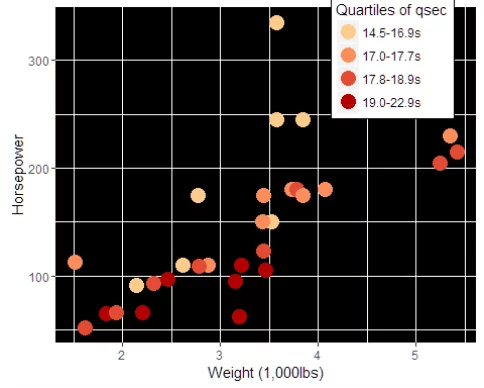This plot provides a first look at the interrelationships of the three variable of interest. To get a different representation of these relations, we use contour plots.

Preparing the Data for Contour Plots in Base R

The contour function requires three dimensional data as an input. We are interested in estimating acceleration for all possible combinations of weight and horsepower using the available data, thereby generating three dimensional data. To compute the estimates, a two-dimensional loess model is fit to the data using the following call:

data.loess <- loess(qsec ~ wt * hp, data = mtcars)

The model contained within the resulting loess object is then used to output the three-dimensional dataset needed for plotting. We do that by generating a sequence of values with uniform spacing over the range of wt and hp. An arbitrarily chosen distance of 0.3 between sequence elements was used to give a relatively fine resolution to the data. Using the predict function, the loess model object is used to estimate a qsec value for each combination of values in the two sequences. These estimates are stored in a matrix where each element of the wt sequence is represented by a row and each element of the hp sequence is represented by a column.

# Create a sequence of incrementally increasing (by 0.3 units) values for both wt and hp
xgrid <-  seq(min(mtcars\$wt), max(mtcars\$wt), 0.3)
ygrid <-  seq(min(mtcars\$hp), max(mtcars\$hp), 0.3)
# Generate a dataframe with every possible combination of wt and hp
data.fit <-  expand.grid(wt = xgrid, hp = ygrid)
# Feed the dataframe into the loess model and receive a matrix output with estimates of
# acceleration for each combination of wt and hp
mtrx3d <-  predict(data.loess, newdata = data.fit)
# Abbreviated display of final matrix
mtrx3d[1:4, 1:4]

##           hp
## wt         hp= 52.0 hp= 52.3 hp= 52.6 hp= 52.9
##   wt=1.513 19.04237 19.03263 19.02285 19.01302
##   wt=1.813 19.25566 19.24637 19.23703 19.22764
##   wt=2.113 19.55298 19.54418 19.53534 19.52645
##   wt=2.413 20.06436 20.05761 20.05077 20.04383

We then visualize the resulting three dimensional data using the contour function.

contour(x = xgrid, y = ygrid, z = mtrx3d, xlab = “Weight (1,000lbs)”, ylab = “Horsepower”)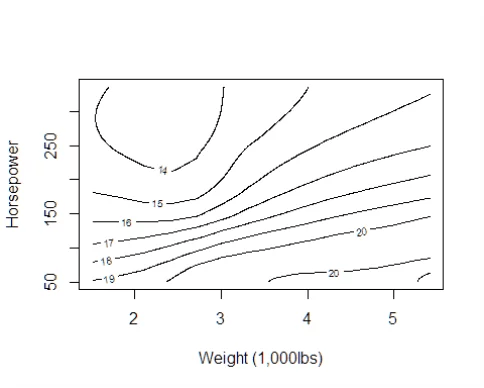Preparing the Data for Contour Plots in GGPlots

To use ggplot, we manipulate the data into “long format” using the melt function from the reshape2 package. We add names for all of the resulting columns for clarity. An unfortunate side effect of the predict function used to populate the initial 3d dataset is that all of the row values and column values of the resulting matrix are of type char, in the form of “variable = value“. The character portion of these values need to first be removed then the remaining values converted to numeric. This is done using str_locate (from the stringR package) to locate the “=” character, then use str_sub (also from stringR) to extract only the numerical portion of the character string. Finally, as.numeric is used to convert results to the appropriate class.

# Transform data to long form
mtrx.melt <- melt(mtrx3d, id.vars = c(“wt”, “hp”), measure.vars = “qsec”)
names(mtrx.melt) <- c(“wt”, “hp”, “qsec”)
# Return data to numeric form
mtrx.melt\$wt <- as.numeric(str_sub(mtrx.melt\$wt, str_locate(mtrx.melt\$wt, “=”)[1,1] + 1))
mtrx.melt\$hp <- as.numeric(str_sub(mtrx.melt\$hp, str_locate(mtrx.melt\$hp, “=”)[1,1] + 1))

##      wt hp     qsec
## 1 1.513 52 19.04237
## 2 1.813 52 19.25566
## 3 2.113 52 19.55298
## 4 2.413 52 20.06436
## 5 2.713 52 20.65788
## 6 3.013 52 20.88378

Using GGPlots2 to Create Contour Plots

Basic Contour Plot

With the data transformed into “long” form, we can make contour plots with ggplot2. With the most basic parameters in place, we see:

plot1 <- ggplot(mtrx.melt, aes(x = wt, y = hp, z = qsec)) +
stat_contour()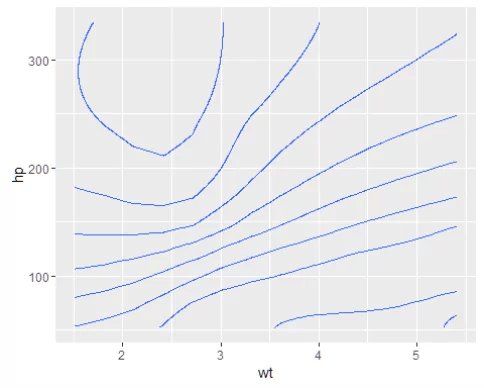The resulting plot is not very descriptive and has no indication of the values of qsec.

Contour plot with plot region colored using a continuous outcome variable (qsec).

To aid in our plot’s descriptive value, we add color to the contour plot based on values of qsec.

plot2 <- ggplot(mtrx.melt, aes(x = wt, y = hp, z = qsec)) +
stat_contour(geom = “polygon”, aes(fill = ..level..)) +
geom_tile(aes(fill = qsec)) +
stat_contour(bins = 15) +
xlab(“Weight (1,000lbs)”) +
ylab(“Horsepower”) +
guides(fill = guide_colorbar(title = “¼ Mi. Time (s)”))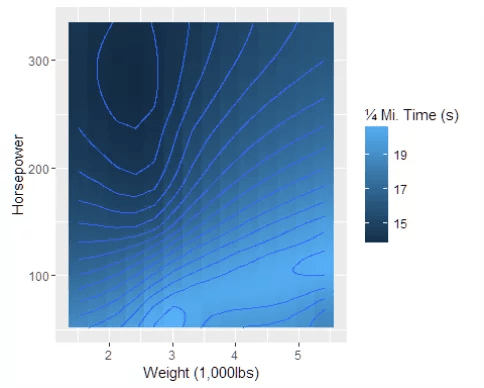Contour plot with plot region colored using discrete levels

Another option could be to add colored regions between contour lines. In this case, we will split qsec into 10 equal segments using the cut function.

# Create ten segments to be colored in
mtrx.melt\$equalSpace <- cut(mtrx.melt\$qsec, 10)
# Sort the segments in ascending order
breaks <- levels(unique(mtrx.melt\$equalSpace))
# Plot
plot3 <- ggplot() +
geom_tile(data = mtrx.melt, aes(wt, hp, qsec, fill = equalSpace)) +
geom_contour(color = “white”, alpha = 0.5) +
theme_bw() +
xlab(“Weight (1,000lbs)”) +
ylab(“Horsepower”) +
scale_fill_manual(values = c(“#35978f”, “#80cdc1”, “#c7eae5”, “#f5f5f5”,
“#f6e8c3”, “#dfc27d”, “#bf812d”, “#8c510a”,
“#543005”, “#330000”),
name = “¼ Mi. Time (s)”, breaks = breaks, labels = breaks)

## Warning in max(vapply(evaled, length, integer(1))): no non-missing
## arguments to max; returning -InfNote: in the lower right hand corner of the graph above, there is a region where increasing weight is associated with decreasing ¼ mile times, which is not characteristic of the true relation between weight and acceleration. This is due to extrapolation that the predict function performed while creating predictions for qsec for combinations of weight and height that did not exist in the raw data. This cannot be avoided using the methods described above. A well-placed rectangle (geom_rect) or placing the legend over the offending area can conceal this region (see example below).

Contour plot with contour lines colored using a continuous outcome variable (qsec)

Instead of coloring the whole plot, it may be more desirable to color just the contour lines of the plot. This can be achieved by using the stat_contour aesthetic over the scale_fill_manual aesthetic. We also chose to move the legend in the area of extrapolation.

plot4 <- ggplot()  +
theme_bw() +
xlab(“Weight (1,000lbs)”) +
ylab(“Horspower”) +
stat_contour(data = mtrx.melt, aes(x = wt, y = hp, z = qsec, colour = ..level..),
breaks = round(quantile(mtrx.melt\$qsec, seq(0, 1, 0.1)), 0), size = 1) +
scale_color_continuous(name = “¼ Mi. Time (s)”) +
theme(legend.justification=c(1, 0), legend.position=c(1, 0))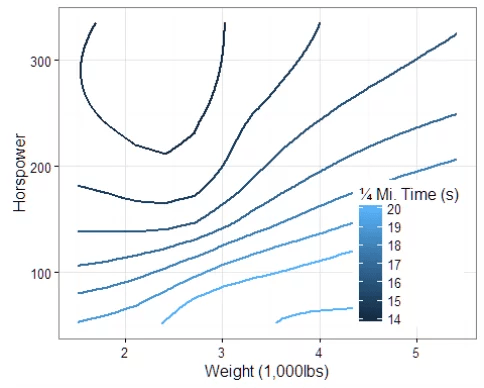Contour plot with contour lines colored using a continuous outcome variable and overlaying scatterplot of weight and horsepower.

We can also overlay the raw data from mtcars onto the previous plot.

plot5 <- plot4 +
geom_point(data = mtcars, aes(x = wt, y = hp), shape = 1, size = 2.5, color = “red”)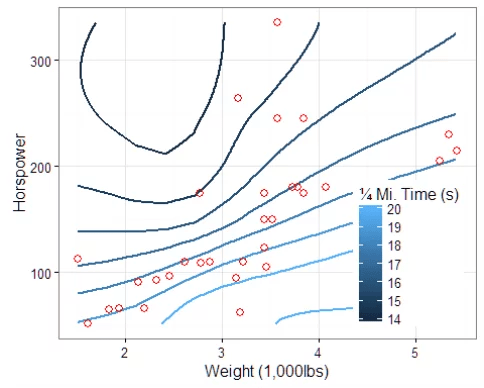Contour plot with contour lines colored using a continuous outcome variable and labeled using direct.labels()

With color-coded contour lines, as seen in the previous example, it may be difficult to differentiate the values of qsec that each line represents. Although we supplied a legend to the preceding plot, using direct.labels from the “directlabels” package can clarify values of qsec.

plot6 <- direct.label(plot5, “bottom.pieces”)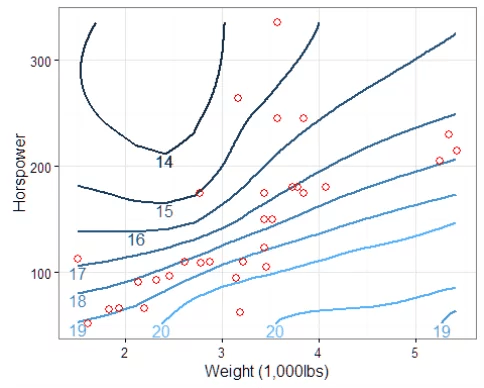We hope that these examples were of help to you and that you are better able to visualize your data as a result.

For questions, corrections, or suggestions for improvement, contact John at [email protected] or using @JohnBellettiere via Twitter.

Posted on Categories Guest Post, R, visualizationTags

## 4 thoughts on “Using 2D Contour Plots within {ggplot2} to Visualize Relationships between Three Variables”

1.c says:

Is this blog posted originally written as a .Rmd file in rstudio? If so, how did you publish it on a wordpress site? RWordPress package no longer works with the XML-RPC api for worpress…. thanks!

1.Tal Galili says:

I did the following trick: I saved it as docx file and then imported it to wordpress using the “Aspose Doc Importer” plugin 🙂
Let me know if it works for you or if you find a better solution…

Tal

This site uses Akismet to reduce spam. Learn how your comment data is processed.